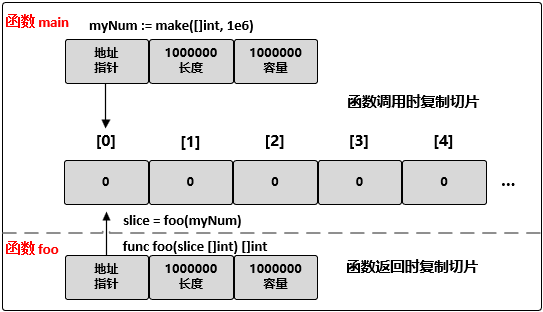# Golang 入门 : 切片(slice) - sparkdev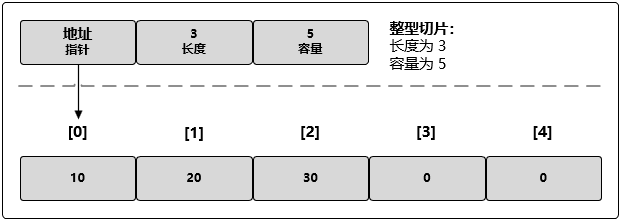// 创建一个整型切片// 其长度和容量都是 5 个元素slice := make([]int, 5)

// 创建一个整型切片// 其长度为 3 个元素，容量为 5 个元素slice := make([]int, 3, 5)

// 创建一个整型切片// 使其长度大于容量myNum := make([]int, 5, 3)

// 创建字符串切片// 其长度和容量都是 3 个元素myStr := []string{"Jack", "Mark", "Nick"}// 创建一个整型切片// 其长度和容量都是 4 个元素myNum := []int{10, 20, 30, 40}

// 创建字符串切片// 使用空字符串初始化第 100 个元素myStr := []string{99: ""}

// 创建有 3 个元素的整型数组myArray := int{10, 20, 30}// 创建长度和容量都是 3 的整型切片mySlice := []int{10, 20, 30}nil 和空切片

// 创建 nil 整型切片var myNum []int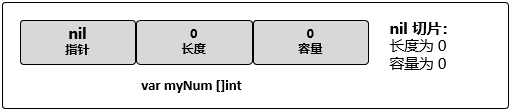// 使用 make 创建空的整型切片myNum := make([]int, 0)// 使用切片字面量创建空的整型切片myNum := []int{}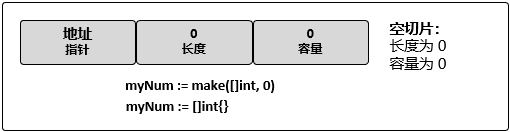// 创建一个整型切片// 其容量和长度都是 5 个元素myNum := []int{10, 20, 30, 40, 50}// 改变索引为 1 的元素的值myNum  = 25通过切片创建新的切片

slice[i:j]slice[i:j:k]

slice[i:] // 从 i 切到最尾部slice[:j] // 从最开头切到 j(不包含 j)slice[:] // 从头切到尾，等价于复制整个 slice

// 创建一个整型切片// 其长度和容量都是 5 个元素myNum := []int{10, 20, 30, 40, 50}// 创建一个新切片// 其长度为 2 个元素，容量为 4 个元素newNum := slice[1:3]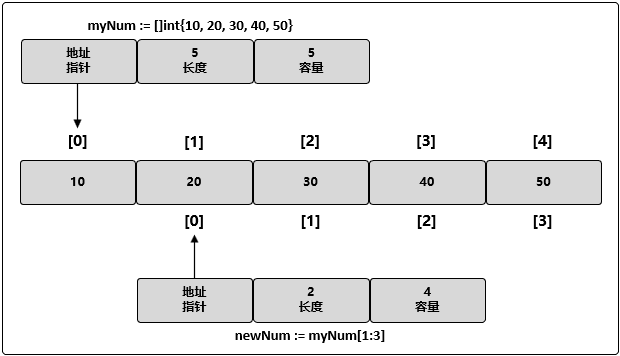// 修改 newNum 索引为 1 的元素// 同时也修改了原切片 myNum 的索引为 2 的元素newNum = 35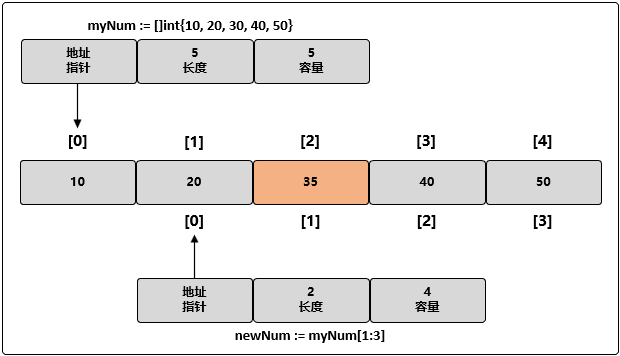// 修改 newNum 索引为 3 的元素// 这个元素对于 newNum 来说并不存在newNum = 45

myNum := []int{10, 20, 30, 40, 50}// 创建新的切片，其长度为 2 个元素，容量为 4 个元素newNum := myNum[1:3]// 使用原有的容量来分配一个新元素// 将新元素赋值为 60newNum = append(newNum, 60)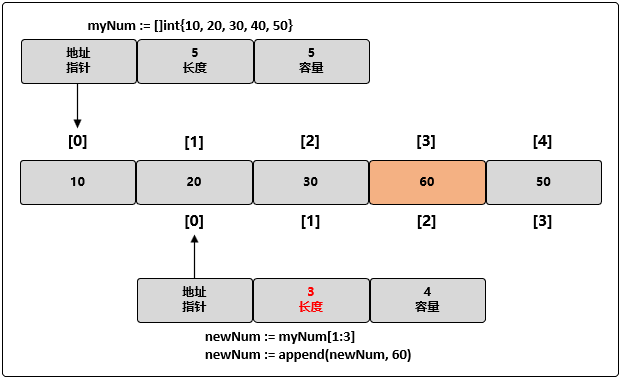// 创建一个长度和容量都是 4 的整型切片myNum := []int{10, 20, 30, 40}// 向切片追加一个新元素// 将新元素赋值为 50newNum := append(myNum, 50)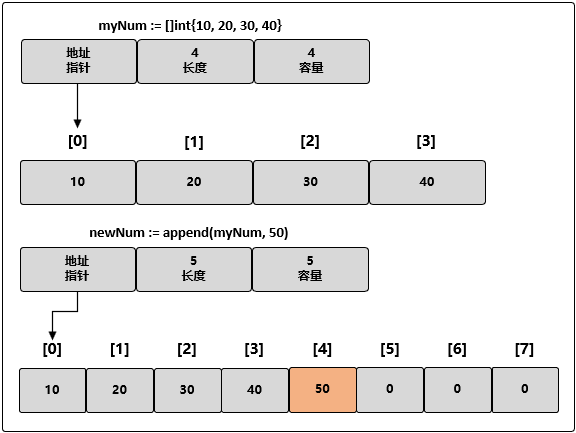// 创建长度和容量都是 5 的字符串切片fruit := []string{"Apple", "Orange", "Plum", "Banana", "Grape"}

// 将第三个元素切片，并限制容量// 其长度为 1 个元素，容量为 2 个元素myFruit := fruit[2:3:4]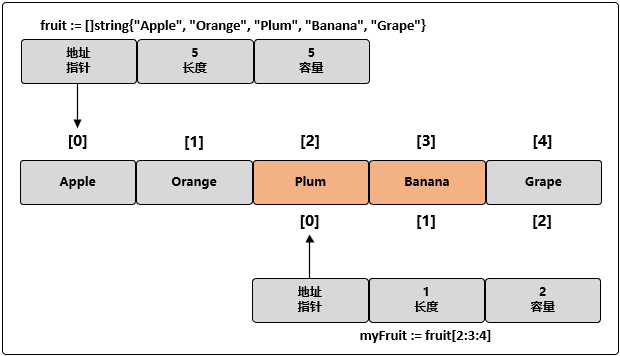myFruit := fruit[2:3:3]// 向 myFruit 追加新字符串myFruit = append(myFruit, "Kiwi")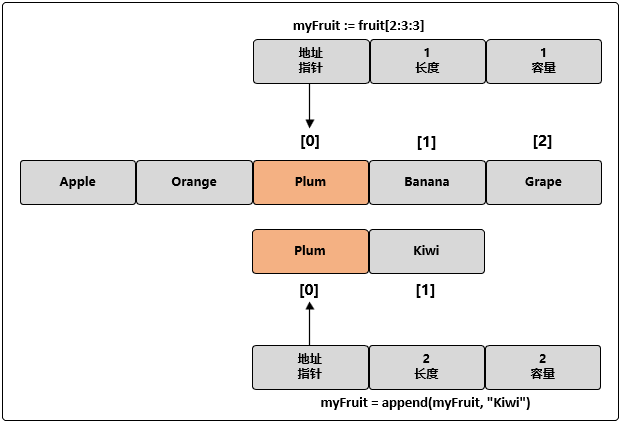// 创建两个切片，并分别用两个整数进行初始化num1 := []int{1, 2}num2 := []int{3, 4}// 将两个切片追加在一起，并显示结果fmt.Printf("%v\n", append(num1, num2...))

myNum := []int{10, 20, 30, 40, 50}// 迭代每一个元素，并显示其值for index, value := range myNum { fmt.Printf("index: %d value: %d\n", index, value)}

index: 0 value: 10index: 1 value: 20index: 2 value: 30index: 3 value: 40index: 4 value: 50

myNum := []int{10, 20, 30, 40, 50}// 修改切片元素的值// 使用空白标识符(下划线)来忽略原始值for index, _ := range myNum { myNum[index] += 1}for index, value := range myNum { fmt.Printf("index: %d value: %d\n", index, value)}

index: 0 value: 11index: 1 value: 21index: 2 value: 31index: 3 value: 41index: 4 value: 51

myNum := []int{10, 20, 30, 40, 50}// 从第三个元素开始迭代每个元素for index := 2; index < len(myNum); index++ { ...}切片间的拷贝操作

Golang 内置的 copy() 函数可以将一个切片中的元素拷贝到另一个切片中，其函数声明为：

func copy(dst, src []Type) int

num1 := []int{10, 20, 30}num2 := make([]int, 5)count := copy(num2, num1)fmt.Println(count)fmt.Println(num2)

3[10 20 30 0 0]

3 表示拷贝成功的元素个数。

myNum := make([]int, 1e6)// 将 myNum 传递到函数 foo()slice = foo(myNum)// 函数 foo() 接收一个整型切片，并返回这个切片func foo(slice []int) []int {...return slice}